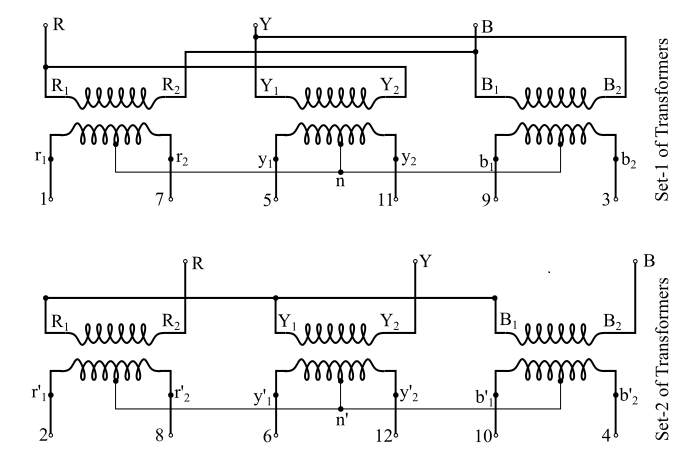# Three-Phase to Twelve-Phase Transformers: Circuit Diagram and Phasor Diagram

The circuit arrangement of the transformers for three-to-twelve phase transformation is shown in the figure.Here, two banks of three 1-phase transformers or two 3-phase transformers are required. In this connection, the secondary windings are arranged in the form of pair of double-star connections. The primary windings of one set of transformers or of one of 3-phase transformers being connected in star while the primary windings of the other being connected in delta. Hence, the arrangement can be named as star-delta / double-star connection for transforming 3-phase to 12-phase. The phasor diagrams of both sets of transformers are shown in the figure.There is a phase shift of 30° between the secondary star voltages of the two six phase systems constituting a balanced 12-phase system. Here, the marking of the secondary terminals can be with the help of figure shown below.Now, if V1 be the input line voltage, then

$$\mathrm{Phase\:Voltage\:of\:star\:connected \:priamry =\frac{𝑉_{1}}{√3}}$$

And

$$\mathrm{Phase\:Voltage\:of\:delta\:connected \:priamry = 𝑉_{1}}$$

Also, if V2 be the line voltage on the secondary side, where (refer the figure above)

$$\mathrm{𝑉_{2} = length\:of\:phasor\:𝑦_{2}{𝑏^′}_{1}\:etc.}$$

Then, from ΔOMy2,

$$\mathrm{\frac{𝑀𝑦_{2}}{𝑂𝑦_{2}}= sin\:15°}$$

$$\mathrm{\Rightarrow\:\frac{𝑉_{2}/2}{𝑂𝑦_{2}}= sin\:15°}$$

$$\mathrm{\Rightarrow\:𝑂𝑦_{2}=\frac{𝑉_{2}}{2\:sin\:15°}=1.932\:𝑉_{2}}$$

Hence, the line to neutral voltage on the secondary side is 1.932 times of secondary line voltage (V2).

If the secondary windings are provided with the mid-tap points, then they should be designed for a voltage of 2 × 1.932 V2 = 3.864 V2.

In order to provide a path for third harmonic currents, the transformer with the star connected primary winding, must have a tertiary winding connected in delta.

As the neutral point is present, hence, the star-delta / double star connection can be used for rectifier and inverter circuits.

Updated on: 14-Aug-2021

543 Views